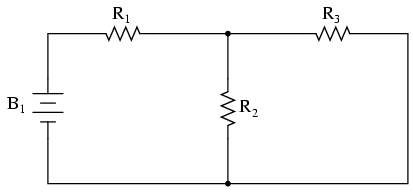� Experiment � Calculator/Converters � Radio � Newsletter � Associations and Societies � Component Manufacturers

 Electronics Symentics A B C D E F G H I J K L M N O P Q R S T U V W X Y Z

 DC Network Analysis

 Generally speaking, network analysis is any structured technique used to mathematically analyze a circuit (a "network" of interconnected components). Quite often the technician or engineer will encounter circuits containing multiple sources of power or component configurations which defy simplification by series/parallel analysis techniques. In those cases, he or she will be forced to use other means. This chapter presents a few techniques useful in analyzing such complex circuits. To illustrate how even a simple circuit can defy analysis by breakdown into series and parallel portions, take start with this series-parallel circuit:To analyze the above circuit, one would first find the equivalent of R2 and R3 in parallel, then add R1 in series to arrive at a total resistance. Then, taking the voltage of battery B1 with that total circuit resistance, the total current could be calculated through the use of Ohm's Law (I=E/R), then that current figure used to calculate voltage drops in the circuit. All in all, a fairly simple procedure.